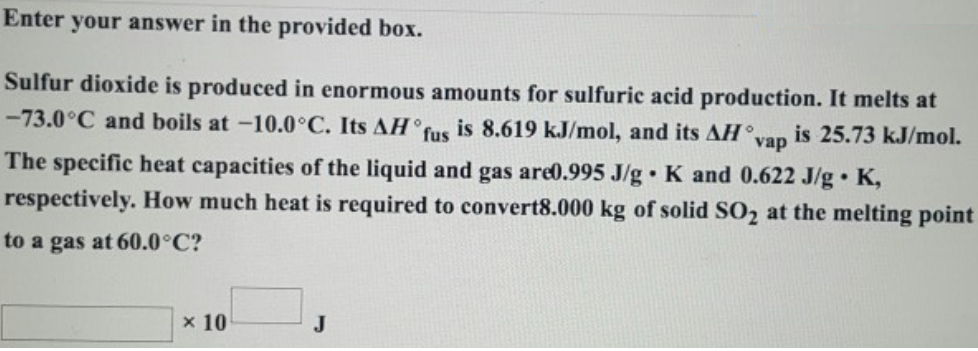# Problem: Enter your answer in the provided box. Sulfur dioxide is produced in enormous amounts for sulfuric acid production. It melts at -73.0°C and boils at -10.0°C. Its ΔH° is 8.619 kJ/mol, and its ΔH°vap is 25.73 kJ/mol. The specific heat capacities of the liquid and gas are 0.995 J/g • K and 0.622 J/g • K, respectively. How much heat is required to convert 8.000 kg of solid SO2 at the melting point to a gas at 60.0°C?

###### FREE Expert Solution
93% (15 ratings)###### Problem Details

Sulfur dioxide is produced in enormous amounts for sulfuric acid production. It melts at -73.0°C and boils at -10.0°C. Its ΔH° is 8.619 kJ/mol, and its ΔH°vap is 25.73 kJ/mol. The specific heat capacities of the liquid and gas are 0.995 J/g • K and 0.622 J/g • K, respectively. How much heat is required to convert 8.000 kg of solid SO2 at the melting point to a gas at 60.0°C?